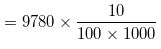Newbie

# In one fortnight of a given month, there was a rainfall of 10 cm in a river valley. If the area of the valley is 97280 km2, show that the total rainfall was approximately equivalent to the addition to the normal water of three rivers each 1072 km long, 75 m wide and 3 m deep. Q.4

• 0

How i solve the ncert class 1oth question of chapter surface areas and volumes give me the easiest solution of the exercise 13.5 question number 4 . Find the best solution of this question and also find the easiest solution of this question. In one fortnight of a given month, there was a rainfall of 10 cm in a river valley. If the area of the valley is 97280 km2, show that the total rainfall was approximately equivalent to the addition to the normal water of three rivers each 1072 km long, 75 m wide and 3 m deep.

Share

1. From the question, it is clear that

Total volume of 3 rivers = 3×[(Surface area of a river)×Depth]

Given,

Surface area of a river = [1072×(75/1000)] km

And,

Depth = (3/1000) km

Now, volume of 3 rivers = 3×[1072×(75/1000)]×(3/1000)

= 0.72 km3

Now, volume of rainfall = total surface area × total height of rain= 9.7 km3

For the total rainfall was approximately equivalent to the addition to the normal water of three rivers, the volume of rainfall has to be equal to volume of 3 rivers.

But, 9.7 km3 ≠ 0.72 km3

So, the question statement is false.

• 0